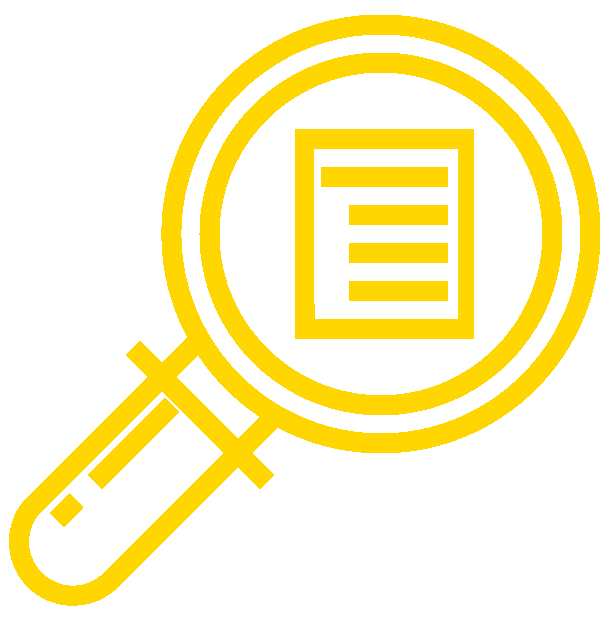Learning OutcomesThe textbook content, assignments, and assessments for Mathematics for Liberal Arts are aligned to the following learning outcomes. See the list of detailed learning outcomes here: Detailed Learning Outcomes: Math for Liberal Arts Corequisite.

Module 1: Historical Counting Systems

• Review topics needed for success in Historical Counting Systems
• Convert between our number system and early counting systems
• Convert numbers between different bases

Module 2: General Problem Solving

• Review topics needed for success in General Problem Solving
• Apply proportional relationships to problems involving rates, money, or geometry
• Design a pathway for solving complex multi-step problems

Module 3: Measurement

• Review topics needed for success in Measurement
• Make calculations and solve application problems involving units of measurement
• Convert measurements between metric units and U.S. customary units

Module 4: Graph Theory

• Review topics needed for success in Graph Theory
• Describe the elements of a graph
• Find Euler and Hamiltonian paths and circuits within a defined graph

Module 5: Fractals

• Review topics needed for success in Fractals
• Understand fractal shapes and the scaling dimension relation
• Use complex numbers to generate terms in a recursive relation

Module 6: Theory and Logic

• Review topics needed for success in Set Theory and Logic
• Use proper set notation and Venn diagrams to depict set relations and operations
• Create truth tables to interpret statements and conditionals
• Evaluate arguments using logical inference, truth tables, and Venn diagrams

Module 7: Voting Theory

• Review topics needed for success in Voting Theory
• Determine the winner of a race utilizing various types of voting methods
• Evaluate the fairness of the approval voting method

Module 8: Growth Models

• Review topics needed for success in Growth Models
• Make calculations and predictions using recursive and explicit equations for both linear and exponential growth
• Use the properties of logarithms to solve problems involving logistic growth

Module 8QR: Modeling Growth

• Review topics needed for success in Modeling Growth
• Use a spreadsheet to create scatterplots and analyze trendlines
• Perform linear regression and make calculations on data from real-world situations
• Perform exponential regression and compare with linear regression

Module 9: Finance

• Review topics needed for success in Finance
• Calculate interest rate, time, initial deposit, and total balance of both simple and compound interest situations
• Calculate interest rate, time, balance, payouts, payments, and initial amounts of both annuities and loans

Module 9QR: Financial Literacy

• Review topics needed for success in Financial Literacy
• Use both simple and compound interest equations to compare various real world savings options
• Analyze annuity and loan options by calculating the outcomes of various real-world examples

Module 10: Statistics: Collecting Data

• Review topics needed for success in Collecting Data
• Identify the various aspects of data collection
• Examine the methods for sampling and experimentation and how bias can affect the results

Module 11: Statistics: Describing Data

• Review topics needed for success in Describing Data
• Create and analyze different types of graphs to represent a specific data set
• Summarize a set of numerical data by reporting various measurements

Module 11QR: Statistical Thinking

• Review topics needed for success in Statistical Thinking
• Use spreadsheets to explore measurements of data sets

Module 12: Probability

• Review topics needed for success in Probability
• Calculate the probability of individual and overlapping events in a variety of problems
• Solve counting problems by using permutation and combination equations